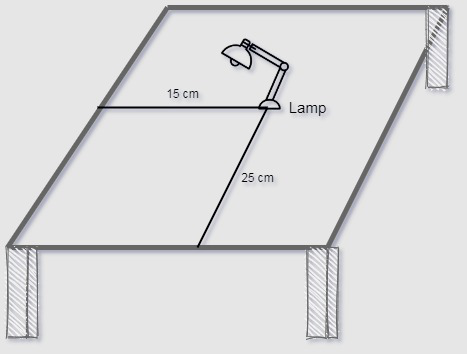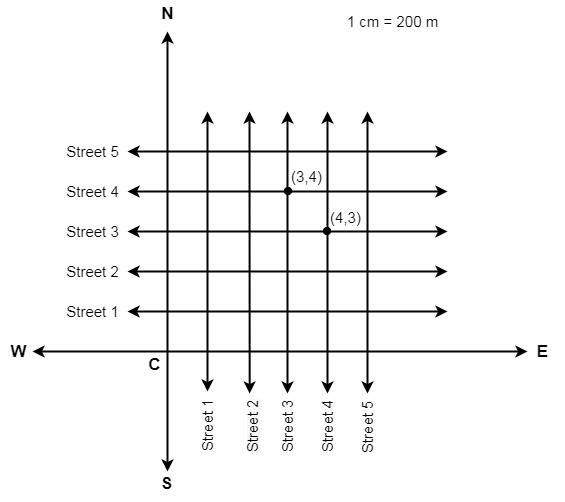Open in App
Not now

## Related Articles

• NCERT Solutions for Class 9 Maths

# Class 9 NCERT Solutions – Chapter 3 Coordinate Geometry – Exercise 3.1

• Last Updated : 23 Feb, 2021

### Question 1: How will you describe the position of a table lamp on your study table to another person?

Solution:• From the figure above consider the position of the lamp as it is a point on a plane of x and y axis.
• Take two line one perpendicular and one horizontal, consider the perpendicular line as Y-axis and horizontal line as X-axis.
• Take the corner of the table as the origin.
• Measure the distance of lamp from Y-axis and X-axis respectively.
• Write the distance in terms of coordinates (15,25).
• Hence we can find the position of lamp on the table.

### Question 2: (Street Plan): A city has two main roads which cross each other at the centre of the city. These two roads are along the North-South direction and East-West direction.All the other streets of the city run parallel to these roads and are 200 m apart. There are 5 streets in each direction. Using 1cm = 200m, draw a model of the city on your notebook. Represent the roads/streets by single lines.There are many cross- streets in your model. A particular cross-street is made by two streets, one running in the North – South direction and another in the East – West direction. Each cross street is referred to in the following manner : If the 2nd street running in the North – South direction and 5th in the East – West direction meet at some crossing, then we will call this cross-street (2, 5). Using this convention, find:(i) how many cross – streets can be referred to as (4, 3).(ii) how many cross – streets can be referred to as (3, 4).

Solution:

• Draw two perpendicular lines that will be referred as the two main roads, mark them as N-S and E-W.
• Take the scale as 1 cm = 200 m as given.
• Mark the intersection of the main road as point C.
• Draw 5-5 parallel line to both the main roads.(i) We can clearly see that there is only one cross street that can be marked as (3,4).
(ii) We can clearly see that there is only one cross street that can be marked as (4,3).

My Personal Notes arrow_drop_up